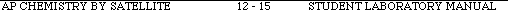### ContentsReturn toIndex of ExperimentsPre-lab QuestionsExperimentPost-lab Questions

EXPERIMENT 12: KINETICS OF THE DECOLORIZATION OF CRYSTAL VIOLET BY SODIUM HYDROXIDE

The following preparatory questions should be answered before coming to class. They are intended to introduce you to several ideas important to aspects of the experiment. You must turn in your work to your instructor before you will be allowed to begin the experiment. Be sure to bring a calculator and paper to laboratory.

1. A certain chemical reaction may be represented by the equation: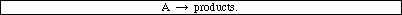The concentration of compound A was measured at several time intervals after the reaction had begun. The three plots, two shown below and one on the following page were constructed using that data. A "best fit" straight line was drawn for each plot. What is the reaction order for this reaction? Explain how you were able to arrive at your answer.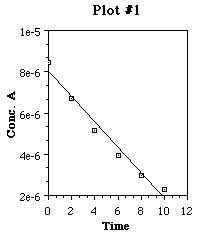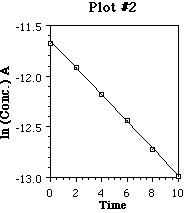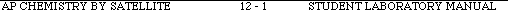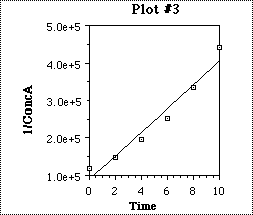2. (a) Write the chemical equation which describes the reaction between crystal violet and sodium hydroxide.(b) A solution is prepared by mixing 10.0 mL of 1.0 x 10-4 M crystal violet with 10.0 mL of 0.10 M sodium hydroxide. The mixture is dilutedwith deionized water to a final volume of 100.0 mL. Which compound will be the limiting reagent in the chemical reaction between the crystal violet and the sodium hydroxide?(c) After the reaction has gone to completion, calculate the concentration of all species represented in the chemical equation.(d) How does the concentration of sodium hydroxide change over the course of the reaction?(e) Explain you answer to part (d) by comparing the initial concentrations of the reactants and commenting on how much of each one is consumed in the reaction.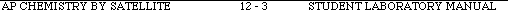EXPERIMENT 12: KINETICS OF THE DECOLORIZATION OF CRYSTAL VIOLET BY SODIUM HYDROXIDE

INTRODUCTION:

Crystal violet is a brightly colored organic compound which is used as a dye. One common use of the dye is in purple ditto masters used to make the purple copies of printed material often distributed to students. Crystal violet is also used as a pH indicator because its color depends upon the acidity of the solution. It's deep purple color is disappears when it reacts with hydroxide ion. The reaction below represents the process:"R" is used to represent the complicated formula of crystal violet. If you would like to see the correct formula for crystal violet, ask your instructor.

Consider the reaction of crystal violet with excess hydroxide ion. As the reaction progresses, less and less of the ionic form of the crystal violet is present, so the solution's color becomes less and less intense. Because this reaction occurs at a relatively slow rate, we can use the Blocktronic spectrometer to monitor its progress. From absorbance readings we can determine the concentration of crystal violet at various times after the reaction has begun.

The rate law for the decolorization reaction has the form:where
[R+] is the concentration of the colored form of crystal violet expressed in moles/liter
k is the rate constant of the reaction at a particular temperature
n is the order of the reaction with respect to crystal violet
m is the order of the reaction with respect to sodium hydroxide

In this experiment, the reaction order with respect to each reactant and the value of the rate constant at room temperature will be determined.The reaction order with respect to R+ will be determined first. The concentration of sodium hydroxide in the reaction mixture will be much greater than that of the crystal violet. It is so much larger, that even after all of the crystal violet has reacted, the sodium hydroxide concentration has not changed very much.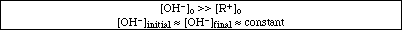The rate law may be rewrittenRate = k' [R+]nEq. 2where k' is a new constant having the valuek' = k [OH-]mEq. 3

To determine the reaction order, the concentration of the crystal violet is followed over a period of time. The Blocktronic spectrometer is used to monitor the concentration of the crystal violet as it changes during the reaction. The reaction order with respect to crystal violet can then be determined graphically using integrated forms of the rate law for a zero, first or second order reaction.

If the reaction is zero order (n = 0) with respect to crystal violet, then[R+]t = -k't + [R+]oPlotting [R+] vs. t (time) yields a straight line with a slope = -k'.

If the reaction is first order (n = 1) with respect to crystal violet, thenln [R+]t = -k't + ln[R+]oPlotting ln[R+] vs. t (time) yields a straight line with a slope = -k'.

If the reaction is second order (n = 2) with respect to crystal violet, thenPlotting 1/[R+] vs. t (time) yields a straight line with a slope = +k'.Once the order of the reaction is determined, the slope of the line is calculated to obtain k'. Recall k' is not a true constant. Its value depends on the initial hydroxide ion concentration, according to Eq. 3. We will measure k' at two different hydroxide ion concentrations, [OH-]A and [OH-]B. We can then write:k'A = k[OH-]AmEq. 4
k'B = k[OH-]Bm.Since k'A, k'B, [OH-]A, and [OH-]B are known values and k and m are constants, we have a system of two equations with two unknowns. This can easily be solved to give the values of k and m.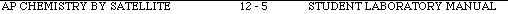(Hint: It helps to use logarithms -)

ln k'A = ln k + m ln[OH-]AEq. 5
ln k'B = ln k + m ln[OH-] B

subtract B from Ak can be calculated by substituting values into either equation in Eq. 4 or Eq. 5.)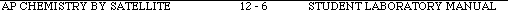EQUIPMENT: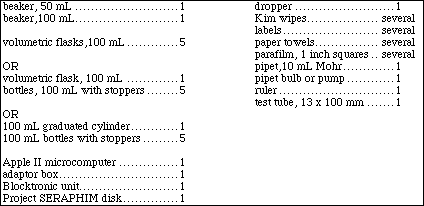PART I: Standard CurveObtain about 60 mL of 1.00 x 10-4 M crystal violet stock solution in a 100 mL beaker. Caution!! Crystal violet will stain hands and clothing!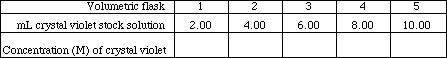Show a sample calculation of the concentration of crystal violet in one of the cylinders.

Calc. #1Connect the Blocktronic unit to the Apple II computer as directed in Appendix II, Section I. Start running the software as directed in Section II.

Calibrate the Blocktronic spectrometer using deionized water as the blank solution. (Refer to Appendix II, Section III as needed for complete instructions.)

Measure the absorbance of the crystal violet solutions (Refer to Appendix II, Section IV as needed for complete instructions) and record the values in Table I.

Table IPrepare a plot of absorbance (y-axis) vs. concentration of crystal violet (x-axis) on the sheet of graph paper provided. Use a ruler to draw the best straight line through the six points. Calculate the slope and y-intercept of the line. Show your work:

Calc. #2Explain how you can use this plot to determine the concentration of an unknown solution of crystal violet.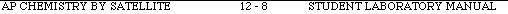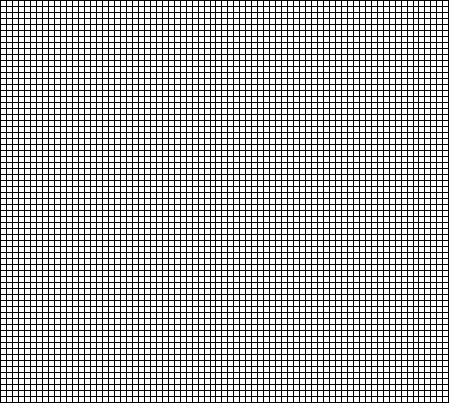EXPERIMENT 12: KINETICS OF THE DECOLORIZATION OF CRYSTAL VIOLET BY SODIUM HYDROXIDE

EQUIPMENT: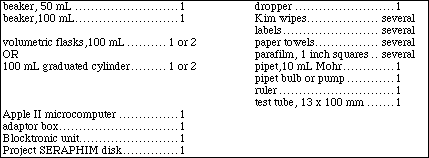PART II: Kinetics Measurement

In this part of the experiment we will be using option 4 from the Blocktronic Main Menu , "Sample Light Levels" for the first time. Review Appendix II, Section V before you start to work.

RUN AUsing a clean pipet, transfer 10.00 mL of 1.00 x 10-4 M crystal violet stock solution into a clean 100 mL volumetric flask. Add about 75 mL of deionized water. Obtain about 20 mL of 0.1 M sodium hydroxide solution in a 50 mL beaker. Carefully clean the pipet with deionized water and then rinse it with a small amount of the sodium hydroxide solution.

Calibrate the Blocktronic using deionized water as the blank. When a suitable blank reading has been obtained, return to the Blocktronic Main Menu and select option 4, Sample Light Levels, by typing "4". When asked, enter "60" seconds for time between samples and hit RETURN. From the display format, choose 1, tabular display by typing "1". For the number of samples, type "11" followed by RETURN.

Read the following paragraph carefully before proceeding:

Working as quickly as possible: Pipet 10.00 mL of 0.1 M sodium hydroxide into the flask. Fill the flask to the mark with deionized water. Stopper and invert the flask 2 or 3 times to mix the solution. Rinse the test tube with a few milliliters of solution. Dispose of the rinsing and fill the test tube 3/4 full of solution. Wipe the outside of the test tube and place it in the Blocktronic unit being careful to line up the mark on the test tube with the mark on the Blocktronic. Cover with the light shield and begin taking readings by pressing the RETURN key. Consider the first reading to be time = 0 minutes. Record the absorbance readings obtained in the table below.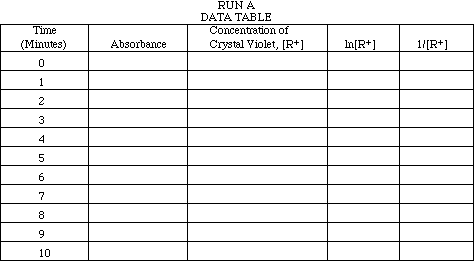When the run is completed and all data has been recorded, follow the instructions on the screen to return to the Main Menu. It is not necessary to save the data unless your instructor tells you to do so.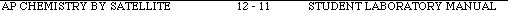RUN B

Review Appendix II, Section V before you start to work.Using a clean pipet, transfer 10.00 mL of 1.00 x 10-4 M crystal violet stock solution into a clean 100 mL volumetric flask. Add about 75 mL of deionized water. Obtain about 20 mL of 0.1 M sodium hydroxide solution in a 50 mL beaker. Carefully clean the pipet with deionized water and rinse it with a small amount of the sodium hydroxide solution.

Calibrate the Blocktronic using deionized water as the blank. When a suitable blank reading has been obtained, return to the Blocktronic Main Menu and select option 4, Sample Light Levels, by typing "4". When asked, enter "60" seconds for time between samples and hit RETURN. From the display format, choose 1, tabular display by typing "1". For the number of samples, type "11" followed by RETURN.

Read the following paragraph carefully before proceeding:

Working as quickly as possible: Pipet 5.00 mL of 0.1 M sodium hydroxide into the flask. Fill the flask to the mark with deionized water. Stopper and invert the flask 2 or 3 times to mix the solution. Rinse the test tube with a few milliliters of solution. Dispose of the rinsing and fill the test tube 3/4 full of solution. Wipe the outside of the test tube and place it in the Blocktronic unit being careful to line up the mark on the test tube with the mark on the Blocktronic. Cover with the light shield and begin taking readings by pressing the RETURN key. Consider the first reading to be time = 0 minutes. Record the absorbance readings obtained in the table below.

RUN B
DATA TABLE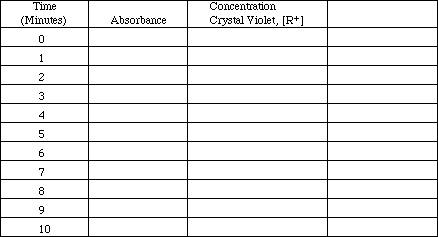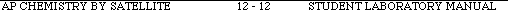Calculations and Post-lab Questions:Top

1. Complete RUN A DATA TABLE. Use the calibration curve obtained in Part I to find the concentration of crystal violet at each time. Show your work for time = 5 minutes as an example.2. Make plots of concentration ([R+]) vs. time, ln[R+] vs. time, and 1/[R+] vs. time for the Run A data on the three sheets of graph paper at the end of the experiment. Which plot is most nearly linear?3. What is the reaction order with respect to crystal violet concentration?4. Complete RUN B DATA TABLE. Find the crystal violet concentration [R+] as in A. Select the appropriate plotting variable, i.e., the one that gives a straight line when plotted against time. Write the symbol for this value ([R+], ln[R+], or 1/[R+]) in the heading of the last column in Table B and fill in the values.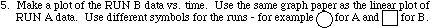6. Use a ruler to draw the best straight line through each set of data. Calculate the slope of each line. Show your work.7. Calculate the values of k'A and k'B.8. Calculate [OH-]A and [OH-]B. Show your work.9. Set up the simultaneous equations described in the introduction and solve for m and k. (Round the exponent to the nearest whole number.) Show your work.10. Write the reaction rate law showing all values (k, n and m).11. What is the overall reaction order?12. Calculate the initial reaction rates for RUN A and for RUN B. How do the two rates compare?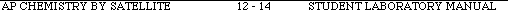### ContentsReturn toIndex of ExperimentsPre-lab QuestionsExperimentPost-lab QuestionsTop